# Engineering Mechanics MCQ Quiz - Objective Question with Answer for Engineering Mechanics - Download Free PDF

Last updated on Sep 12, 2022

## Latest Engineering Mechanics MCQ Objective Questions

#### Engineering Mechanics MCQ Question 1:

The maximum change in entropy is observed during mixing of :

1. 8.6 g hexane + 10.0 g heptane
2. 8.6 g hexane + 5.0 g heptane
3. 4.3 g hexane + 10.0 g heptane
4. 1 g hexane + 10.0 g heptane

Option 1 : 8.6 g hexane + 10.0 g heptane

#### Engineering Mechanics MCQ Question 1 Detailed Solution

Concept:

Mole Concept -

•  The quantity of one mole of a substance signifies 6.022 × 1023 particles of that substance which may be atoms, molecules, or ions.
• The quantity is a universal constant like Dozen, Gross, etc., and is known as the Avogadro number, denoted by NA. after the scientist Amedeo Avogadro.
• Examples- In one mole of H2, there are 6.022 × 1023 molecules of hydrogen, and the number of atoms is 2 × 6.022 × 1023, as one molecule of hydrogen contains two-atom each.
• The mass of one mole of a substance is called its Molar Mass (M) or Atomic mass expressed in grams.
• The volume occupied by a mole of gas is 22.4 L at NTP, called its Molar Volume.
• The no. of moles (n) is calculated as =Number of particles / Avogadro’s number or = Weight of substance/ Molecular Mass of substance.

Entropy:

Entropy refers to the randomness present in the system.

• The value of randomness can be positive or negative, depending on the resultant of the system's randomness.
• The order of randomness is (maximum) Gas > liquid > solid (minimum).
• As, when solid converts to its liquid or gaseous state, the entropy increases, as molecules of the solid-state are packed tightly because of more force of attraction between the molecules, but as it is converted to liquid or the gaseous state, the force of attraction reduces and therefore, the entropy increases.

• If the state on both sides of the reaction, i.e., reactant and product, are the same, the entropy value can be determined by calculating the value of Δng, which refers to the change in the moles of gas molecules.

• Δng = moles of gas in the product – no. of moles of gas in the reactant.

Explanation:

From the above discussion, it is clear that entropy depends upon the quantity of substance.

Among all the options, no. of the moles are maximum in option 1.

Moles of hexane $$=\frac{8.6}{86}=0.1$$

Moles of heptane $$=\frac{10}{100}=0.1$$

Hence, the correct option is (1).

#### Engineering Mechanics MCQ Question 2:

The moment of inertia of a triangular section (base b, height h) about centroidal axis parallel to the base,

1. b3h/12
2. bh3/3
3. bh3/36
4. bh3/2

Option 3 : bh3/36

#### Engineering Mechanics MCQ Question 2 Detailed Solution

Concept:

Moment of inertia of a triangle with base b and height h

$${I_{base}} = \frac{{b{h^3}}}{{12}}$$

Parallel axis theorem

• Parallel axis theorem is used to find a moment of inertia about an axis which is at some distance from the centroidal axis and parallel to centroidal.
• Suppose an axis is at distance d from the centroidal axis and parallel to the centroidal axis.

According to the parallel axis theorem, the moment of inertia about that axis is given by,

$${I_{about~axis~parallel~to~centroida~laxis}} = {I_{about~centroidal~axis}} + A{d^2}$$

Moment of inertia about the centroidal axis of the triangleApplying parallel axis theorem we get

$${I_{xx}} = {I_{cc}} + A{\left( {\frac{h}{3}} \right)^2}$$

$${I_{cc}} = {I_{xx}} - A{\left( {\frac{h}{3}} \right)^2}$$

$$I_{cc}=\frac{{b{h^3}}}{{12}} - \frac{{bh}}{2} \times \frac{{{h^2}}}{9} = \frac{{b{h^3}}}{{36}}$$

$${I_{centroid}} = \frac{{b{h^3}}}{{36}}$$

#### Engineering Mechanics MCQ Question 3:

The forces which meet at one point but their lines of action do not lie in one plane

1. coplanar concurrent forces
2. coplanar non-concurrent forces
3. non-coplanar concurrent forces
4. non-coplanar non-concurrent forces

Option 3 : non-coplanar concurrent forces

#### Engineering Mechanics MCQ Question 3 Detailed Solution

Explanation:

When two or more forces act on a body, they are called to form a system of forces.

Coplanar forces:

• The forces, whose lines of action lie on the same plane, are known as coplanar forces.

Colinear forces:

• The forces, whose lines of action lie on the same line, are known as colinear forces.

Concurrent forces:

• The forces, which meet at one point, are known as concurrent forces. The concurrent forces may or may not be colinear.

Coplanar concurrent forces:

• The forces, which meet at one point and their lines of action also lie on the same plane, are known as coplanar concurrent forces.

Coplanar non-concurrent forces:

• The forces, which do not meet at one point, but their lines of action lie on the same plane, are known as coplanar non-concurrent forces.

Non-coplanar concurrent forces:

• The forces, which meet at one point, but their lines of action do not lie on the same plane, are known as non-coplanar concurrent forces.
• A rigid body is subjected to non-coplanar concurrent force system, $$\rm \sum F_x=\sum F_y=\sum F_z=0$$

Non-coplanar non-concurrent forces:

• The forces, which do not meet at one point and their lines of action do not lie on the same plane, are called non-coplanar non-concurrent forces.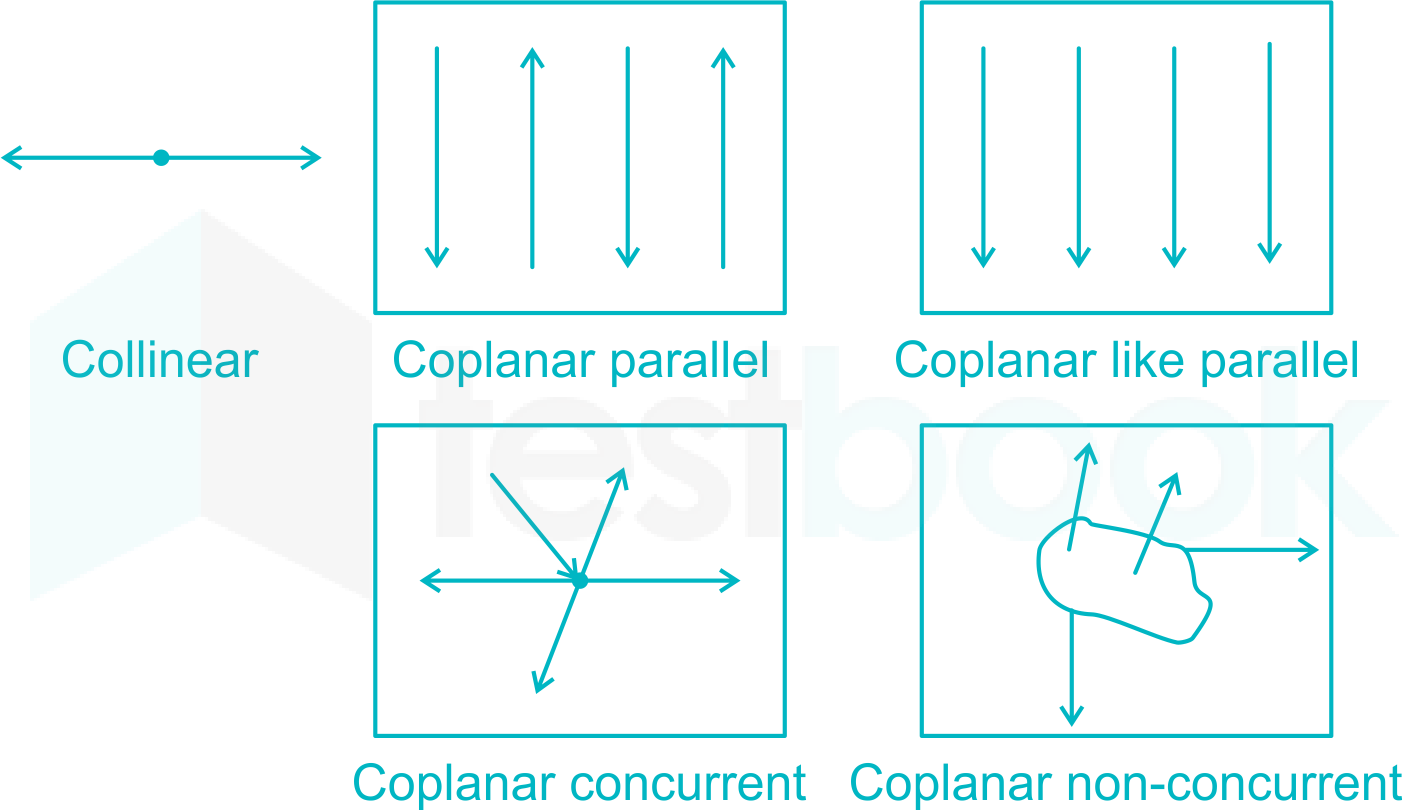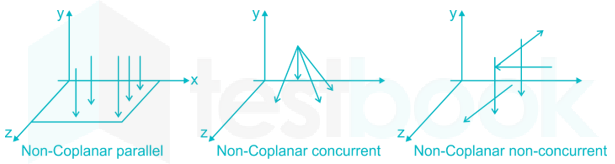#### Engineering Mechanics MCQ Question 4:

The moment of inertia of a rectangular section of width B and depth D about an axis passing through C.G. and parallel to its width is

1. BD2/6
2. BD3/6
3. BD3/12
4. B2D/6

Option 3 : BD3/12

#### Engineering Mechanics MCQ Question 4 Detailed Solution

Explanation: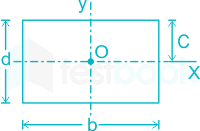Moment of inertia of the rectangular section about X-X axis passing through the C.G. of the section (parallel to width):

$${I_{xx}} = \frac{{b{d^3}}}{{12}}$$

Moment of inertia of the rectangular section about Y-Y axis passing through the C.G. of the section (parallel to depth):

$${I_{yy}} = \frac{{d{b^3}}}{{12}}$$

#### Engineering Mechanics MCQ Question 5:

The section modulus of a circular section at an axis passing its CG is

1. $$\frac{{{\pi d^2}}}{4}$$
2. $$\frac{{\pi {d^2}}}{{16}}$$
3. $$\frac{{\pi {d^3}}}{{16}}$$
4. $$\frac{{\pi {d^3}}}{{32}}$$

Option 4 : $$\frac{{\pi {d^3}}}{{32}}$$

#### Engineering Mechanics MCQ Question 5 Detailed Solution

Explanation:

Sectional Modulus (Z):

It is the ratio of moment of inertia (I) of the beam cross-section about the neutral axis to the distance (ymax) of extreme fiber from the neutral axis.

In the case of a circular section, the section modulus, $$Z = \frac{I}{y_{max}}$$

where,  $$I=\frac{\pi}{64} d^4 = moment~ of ~inertia$$

y = distance from the centroid to top or bottom edge i.e. y = d/2.

$$Z_{cirular\;section}=\frac{I}{y_{max}}$$

$$\therefore~Z_{cirular\;section}=\frac{\frac{\pi{d^4}}{64}}{\frac{d}{2}}$$

$$\therefore~Z_{cirular\;section}=\frac{\pi{d^3}}{32}$$Additional Information

Moment of inertia of different section:

 S.No. Shape of cross-section INA Ymax Z 1 Rectangle $$I = \frac{{b{d^3}}}{{12}}$$ $${Y_{max}} = \frac{d}{2}$$ $$Z = \frac{{b{d^2}}}{6}$$ 2 Triangular $$I = \frac{{B{h^3}}}{{36}}$$ $${Y_{max}} = \frac{{2h}}{3}$$ $$Z = \frac{{B{h^3}}}{{24}}$$

## Top Engineering Mechanics MCQ Objective Questions

#### Engineering Mechanics MCQ Question 6

During inelastic collision of two particles, which one of the following is conserved ?

1. total linear momentum only
2. total kinetic energy only
3. both linear momentum and kinetic energy
4. neither linear momentum nor kinetic energy

Option 1 : total linear momentum only

#### Engineering Mechanics MCQ Question 6 Detailed Solution

Explanation:

• Momentum is conserved in all collisions.
• In elastic collision, kinetic energy is also conserved.
• In inelastic collision, kinetic energy is not conserved. In perfectly inelastic collision, objects stick together after collision.

Perfectly elastic collision:

If law of conservation of momentum and that of kinetic energy hold good during the collision.

Inelastic collision:

If law of conservation of momentum holds good during collision while that of kinetic energy is not.

Coefficient of restitution (e)

$$e = \frac{{Relative\;velocity\;after\;collision}}{{Relative\;velocity\;before\;collision}} = \frac{{{v_2} - {v_1}}}{{{u_1} - {u_2}}}$$

• For perfectly elastic collision, e = 1
• For inelastic collision, e < 1
• For perfectly inelastic collision, e = 0

#### Engineering Mechanics MCQ Question 7

A block weighing 981 N is resting on a horizontal surface. The coefficient of friction between the block and the horizontal surface is μ = 0.2. A vertical cable attached to the block provides partial support as shown. A man can pull horizontally with a force of 100 N. What will be the tension, T (in N) in the cable if the man is just able to move the block to the right?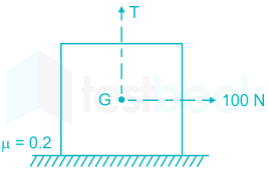1. 176.2
2. 196.0
3. 481.0
4. 981.0

Option 3 : 481.0

#### Engineering Mechanics MCQ Question 7 Detailed Solution

Concept: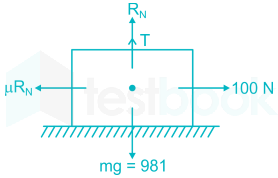Number of vertical forces:

∑Fy = T + RN - W = 0

Static friction force is given as,

Fs = μRN

Calculation:

Given:

W = 981 N, μ = 0.2, Fs = 100 N

Normal reaction:

$$\Rightarrow {R_N} = \frac{{100}}{{0.2}} = 500~N$$

Tension T

⇒ T = 981 - 500 = 481 N

#### Engineering Mechanics MCQ Question 8

The CG of a semicircular plate of 66 cm diameter, from its base, is1. 8/33 cm
2. 1/14 cm
3. 14 cm
4. 63/8 cm

Option 3 : 14 cm

#### Engineering Mechanics MCQ Question 8 Detailed Solution

Concept:

The CG of a semicircular plate of  r radius, from its base, is

$$\bar y = {4r\over 3 \pi}$$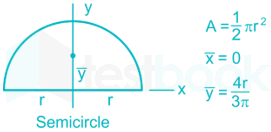Calculation:

Given:

r = 33 cm

$$\bar y = {4r\over 3 \pi}={4\times 33\over3\times{22\over 7}}$$

y̅ = 14 cm

∴ the C.G. of a semicircular plate of 66 cm diameter, from its base, is 14 cm.Additional Information

C.G. of the various plain lamina are shown below in the table. Here x̅  & y̅  represent the distance of C.G. from x and y-axis respectively.

 CircleSemicircleTriangle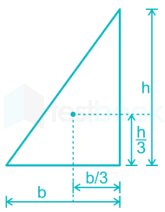Cone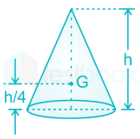Rectangle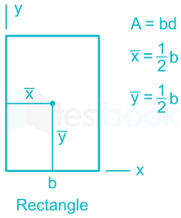Quarter Circle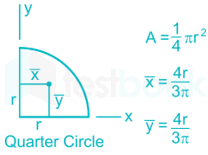Solid hemisphere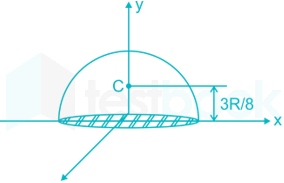#### Engineering Mechanics MCQ Question 9

A particle starts from rest and moves in a straight line whose equation of motion is given by S = 2t3 - t2 - 1. The acceleration of the particle after one second will be-

1. 4 m/s2
2. 6 m/s2
3. 8 m/s2
4. 10 m/s2

Option 4 : 10 m/s2

#### Engineering Mechanics MCQ Question 9 Detailed Solution

Concept:

If s = f(t)

Then, First derivative with respect to time represents the velocity

$$v=\frac{ds}{dt}$$

Acceleration is given by

$$a=\left( \frac{{{d}^{2}}S}{d{{t}^{2}}} \right)$$

Where s is the displacement

Calculation:

Given:

s = 2t3 – t2 - 1 and t = 1 sec.

$$\frac{ds}{dt}=6{{t}^{2}}-2t$$

$$\frac{{{d}^{2}}s}{d{{t}^{2}}}=12t-2$$

$${{\left( \frac{{{d}^{2}}s}{d{{t}^{2}}} \right)}_{t=1s}}=12-2=10 \;m/s^2$$

#### Engineering Mechanics MCQ Question 10

A pin jointed uniform rigid rod of weight W and Length L is supported horizontally by an external force F as shown in the figure below. The force F is suddenly removed. At the instant of force removal, the magnitude of vertical reaction developed at the support is1. zero
2. W/4
3. W/2
4. W

Option 2 : W/4

#### Engineering Mechanics MCQ Question 10 Detailed Solution

Explanation:When the Force F is suddenly removed, then due to W, the rod is in rotating condition with angular acceleration $$\alpha$$

Thus the equation of motion:

$$\Sigma M=I_o\alpha;\;\;W \times \frac{L}{2} = I\alpha$$

$$As, I = \frac{mL^2}{3}= \frac{1}{3}\times\frac{W}{g}\times{L^2}$$

$$\therefore W \times \frac{L}{2} =\frac{WL^2}{3g}\times\alpha$$

$$\Rightarrow \alpha = \frac{{3g}}{{2L}}$$

$$\therefore Linear\;acceleration\;at\;centre = \alpha \times \frac{L}{2} = \frac{{3g}}{{2L}}\times \frac{L}{2}=\frac{{3g}}{4}$$

Also, the centre of the rod accelerates with linear acceleration a;

$$W-R=F\Rightarrow mg-R=ma\\R=mg-ma=mg-\frac{3}{4}mg=\frac{1}{4}mg=\frac{W}{4}$$

#### Engineering Mechanics MCQ Question 11

A thin rod of length L and mass M will have what moment of inertia about an axis passing through one of its edge and perpendicular to the rod?

1. ML2/12
2. ML2/6
3. ML2/3
4. ML2/9

Option 3 : ML2/3

#### Engineering Mechanics MCQ Question 11 Detailed Solution

CONCEPT:

• Parallel axis theorem: Moment of inertia of a body about a given axis I is equal to the sum of moment of inertia of the body about an axis parallel to given axis and passing through centre of mass of the body Io and Ma2, where ‘M’ is the mass of the body and ‘a’ is the perpendicular distance between the two axes.⇒ I = Io + Ma2

EXPLANATION: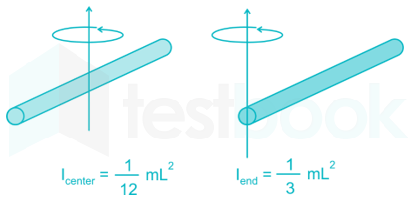• For a uniform rod with negligible thickness, the moment of inertia about its centre of mass is:

$${I_{cm}} = \frac{1}{{12}}M{L^2}$$

Where M = mass of the rod and L = length of the rod

∴ The moment of inertia about the end of the rod is

$$\Rightarrow {I_{end}} = {I_{cm}} + M{d^2}$$

$$\Rightarrow I_{end}= \frac{1}{{12}}M{L^2} + M{\left( {\frac{L}{2}} \right)^2} = \frac{1}{3}M{L^2}$$

#### Engineering Mechanics MCQ Question 12

A force F is given by F = at + bt2 where t is time, what are the dimension of a and b.

1. MLT1, MLT0
2. MLT3, ML2T4
3. MLT-4, MLT-4
4. MLT-3, MLT-4

Option 4 : MLT-3, MLT-4

#### Engineering Mechanics MCQ Question 12 Detailed Solution

CONCEPT:

Principle of homogeneity of dimensions:

• According to this principle, a physical equation will be dimensionally correct if the dimensions of all the terms occurring on both sides of the equation are the same.
• This principle is based on the fact that only the physical quantities of the same kind can be added, subtracted, or compared.
• Thus, velocity can be added to velocity but not to force.

EXPLANATION

Given - F = at + bt2

From the principle of dimensional homogeneity, the left-hand side of the equation dimensionally equal to the right-hand side of the equation.

The dimension formula of force (F) = [MLT-2]

∴ [MLT-2] = [a] [T]

$$⇒ \left[ a \right] = \frac{{\left[ {ML{T^{ - 2}}} \right]}}{{\left[ T \right]}} = \left[ {ML{T^{ - 3}}} \right]$$

For second term,

⇒ [MLT-2] = [b] [T2]

$$\Rightarrow \left[ b \right] = \frac{{\left[ {ML{T^{ - 2}}} \right]}}{{\left[ {{T^2}} \right]}} = \left[ {ML{T^{ - 4}}} \right]$$

#### Engineering Mechanics MCQ Question 13

A rubber ball is thrown vertically upward with a velocity u from the top of a building. It strikes the ground with a velocity 3u. The time taken by the ball to reach the ground is given by:

1. 4u/g
2. 3u/g
3. 2u/g
4. u/g

Option 1 : 4u/g

#### Engineering Mechanics MCQ Question 13 Detailed Solution

Concept:

Equation of motion:

• The mathematical equations used to find the final velocity, displacements, time, etc of a moving object without considering the force acting on it are called equations of motion.
• These equations are only valid when the acceleration of the body is constant and they move in a straight line.

There are three equations of motion:

v = u + at

v2 = u2 + 2as

$$s =ut + \frac{1}{2}at^2$$

where, v = final velocity, u = initial velocity, s = distance travelled by the body under motion, a = acceleration of body under motion, and t = time taken by the body under motion.

Calculation:

Given:

Part-I:

When the ball will reach the highest point then the final velocity will be zero.

Initial velocity = u m/sec, final velocity = 0 m/sec, acceleration = -g m/sec2

applying 1st equation of motion

v = u + at

0 = u - gt1

$$t_1=\frac{u}{g}$$

Part-II:

Initial velocity will be zero as the ball is at the highest point.

applying 1st equation of motion

v = u + at

3u = 0 + gt2

$$t_2=\frac{3u}{g}$$

Therefore total time is:

t = t1 + t2

$$t=\frac{u}{g}+\frac{3u}{g}=\frac{4u}{g}$$

#### Engineering Mechanics MCQ Question 14

A force vs extension graph of a spring is as shown. The work done in extending the spring is1. 10 J
2. 5 J
3. 5000 J
4. 500 J

Option 2 : 5 J

#### Engineering Mechanics MCQ Question 14 Detailed Solution

Concept:

Work done = Area under force deflection diagram

$$Work ~done = \frac 12 \times Force\times deflection$$

Calculation:

Given:

Force = 100 N, deflection = 100 mm = 0.1 m

$$Work ~done = \frac 12 \times Force\times deflection$$

$$Work ~done = \frac 12 \times 100\times 0.1=5~J$$

#### Engineering Mechanics MCQ Question 15

A block of mass 5 kg slides down from rest along a frictionless inclined plane that makes an angle of 30° with horizontal. What will be the speed of the block after it covers a distance of 3.6 m along the plane? [g = 10 m/s2]

1. 5 m/s
2. 6 m/s
3. 7 m/s
4. 8 m/s

Option 2 : 6 m/s

#### Engineering Mechanics MCQ Question 15 Detailed Solution

Concept

The equation of motions are

v = u + at

v2 = u2 + 2as

$$s = ut + \frac{1}{2}a{t^2}$$

Calculation:

Given:

Mass of the block, m = 5 kg, Inclination angle of the plane, θ = 30°, Initial velocity of the block, u = 0 m/s, Distance travelled by the block, s = 3.6 m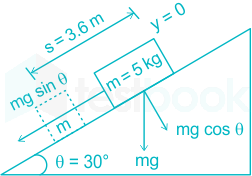Force acting on the block along with the inclination of plane =  $$mg\sin 30^\circ = 5 \times 10 \times \frac{1}{2}\Rightarrow 25\;N$$

Acceleration of the block along the inclined plane, a = $$g\sin 30^\circ = 5~m/{s^2}$$

Applying equation of motion along the inclined plane.

v2 = u2 + 2as

v= 0 + 2 × 5 × 3.6

∴ v = 6 m/s.

#### Engineering Mechanics MCQ Question 16

In a statically determinate plane truss, the number of joints (j) and the number of members (m) are related by

1. j = 2m – 3
2. m = 2j + 1
3. m = 2j – 3
4. m = 2j – 1

Option 3 : m = 2j – 3

#### Engineering Mechanics MCQ Question 16 Detailed Solution

Explanation:

Truss:

• A framework composed of members joined at their ends to form a rigid structure.

Planar truss:

• In these types of trusses, members lie in a single plane.
• The basic element of a planar truss is a triangle i.e. three members are joined at three points.
• For a planar truss, as there are three unknown support reactions (For a node or joint, there will be three unknown but dependent support reactions). so if
1. m = 2j - 3 then system is statically determinate
2. m < 2j - 3 then system is collapsed
3. m > 2j - 3 then system is statically indeterminate
• where, m = number of members, j = total number of joints (including those attached to the supports)

#### Engineering Mechanics MCQ Question 17

A thin disc and a thin ring, both have mass M and radius R. Both rotate about axes through their centre of mass and are perpendicular to their surfaces at the same angular velocity. Which of the following is true?

1. The ring has higher kinetic energy
2. The disc has higher kinetic energy
3. The ring and the disc have the same kinetic energy
4. Kinetic energies of both the bodies are zero since they are not in linear motion

Option 1 : The ring has higher kinetic energy

#### Engineering Mechanics MCQ Question 17 Detailed Solution

CONCEPT:

Moment of inertia:

• The moment of inertia of a rigid body about a fixed axis is defined as the sum of the product of the masses of the particles constituting the body and the square of their respective distances from the axis of the rotation.
• The moment of inertia of a particle  is

⇒ I = mr2

Where r = the perpendicular distance of the particle from the rotational axis.

• Moment of inertia of a body made up of a number of particles (discrete distribution)

⇒ I = m1r12 + m2r22 + m3r32 + m4r42 + -------

Rotational kinetic energy:

• The energy, which a body has by virtue of its rotational motion, is called rotational kinetic energy.
• A body rotating about a fixed axis possesses kinetic energy because its constituent particles are in motion, even though the body as a whole remains in place.
• Mathematically rotational kinetic energy can be written as -

⇒ KE $$= \frac{1}{2}I{\omega ^2}$$

Where I = moment of inertia and ω = angular velocity.

EXPLANATION:

• The moment of inertia of the ring about an axis passing through the center and perpendicular to its plane is given by

⇒ Iring = MR2

• Moment of inertia of the disc about an axis passing through center and perpendicular to its plane is given by -

$$⇒ {I_{disc}} = \frac{1}{2}M{R^2}$$

• As we know that mathematically rotational kinetic energy can be written as

$$⇒ KE = \frac{1}{2}I{\omega ^2}$$

• According to the question, the angular velocity of a thin disc and a thin ring is the same. Therefore, the kinetic energy depends on the moment of inertia.
• Therefore, a body having more moments of inertia will have more kinetic energy and vice - versa.
• So, from the equation, it is clear that,

⇒ Iring > Idisc

∴ Kring > Kdisc

• The ring has higher kinetic energy.Body Axis of Rotation Moment of inertia Uniform circular ring of radius R perpendicular to its plane and through the center MR2 Uniform circular ring of radius R diameter $$\frac{MR^2}{2}$$ Uniform circular disc of radius R perpendicular to its plane and through the center $$\frac{MR^2}{2}$$ Uniform circular disc of radius R diameter $$\frac{MR^2}{4}$$ A hollow cylinder of radius R Axis of cylinder MR2

#### Engineering Mechanics MCQ Question 18

An elevator weighing 1000 kg attains an upward velocity of 4 m/sec in two seconds with uniform acceleration. The tension (in N) in supporting cable will be (g = 9.8 m/s2)

1. 1204 N
2. 9800 N
3. 2000 N
4. 11800 N

Option 4 : 11800 N

#### Engineering Mechanics MCQ Question 18 Detailed Solution

Concept:

Considering the free body diagram of the lift,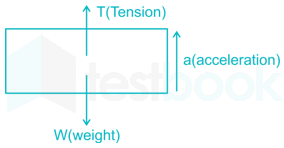Using condition of equili

Force = T - W

ma = T - W ⇒ T = ma + mg

Calculation:

Given:

W = 1000 kg, v = 4 m/s, t = 2 sec

Using equation of motion,

v = u + at

Initaillay, the velocity of elevator is zero.

∴ 4 =  0 + 2a

$$a\; = \;\frac{{4 }}{2}\; = \;2~m/{s^2}$$

Tension is given as

T = mg + ma = m (g + a)

T = 1000 (9.8 + 2)

T = 11800 N

#### Engineering Mechanics MCQ Question 19

A 1 kg block is resting on a surface with coefficient of friction 0.1. A force of 0.8 N is applied to the block as shown in figure. The friction force is1. 0 N
2. 0.8 N
3. 0.98 N
4. 1.2 N

Option 2 : 0.8 N

#### Engineering Mechanics MCQ Question 19 Detailed Solution

Concept:

The friction force is given by:

f = μN

where μ is the coefficient of friction between the surfaces in contact, N is the normal force perpendicular to friction force.

Calculation:

Given:

μ = 0.1, m = 1 kg, F = 0.8 N

Now, we know that

From the FBD as shown below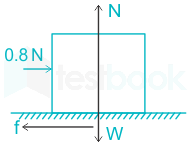Normal reaction, N = mg = 1 × 9.81 = 9.81 N

Limiting friction force between the block and the surface, f = μN =  0.1 × 9.81 = 0.98 N

But the applied force is 0.8 N which is less than the limiting friction force.

∴ The friction force for the given case is 0.8 N.

#### Engineering Mechanics MCQ Question 20

A 5 m long ladder is resting on a smooth vertical wall with its lower end 3 m from the wall. What should be the coefficient of friction between the ladder and the floor for equilibrium?

1. $$\frac{1}{2}$$
2. $$\frac{3}{8}$$
3. $$\frac{1}{3}$$
4. $$\frac{3}{5}$$

Option 2 : $$\frac{3}{8}$$

#### Engineering Mechanics MCQ Question 20 Detailed Solution

Concept:

The resting on between any frictional floor and a vertical wall will always satisfy all the static equilibrium condition i.e.

∑ Fx = ∑ Fy = ∑ Mat any point = 0

Calculation:

Given:

Length of ladder (AB) = 5 m, OB = 3 m

Let W will be the weight of the ladder, NB and NA will be support reaction, θ is the angle between ladder and floor and μ is the friction coefficient between ladder and floor.

Free body diagram of the ladder;OA2 = AB2 - OB, OA2 = 52 - 32

OA2 = 16, OA = 4 m

From Δ OAB,

$$\cos θ = \frac{3}{5}$$

Now apply ∑ Fy = 0

NB = W
Now take moment about point A, which should be equal to zero

∑ M= 0

$$\;\left( {\mu {N_B} \times 4} \right) + \left( {W \times \frac{5}{2} \times \cos \theta } \right) = {N_B} \times 3$$

$$\;\left( {\mu {N_B} \times 4} \right) + \left( {{N_B} \times \frac{5}{2} \times \frac{3}{5}} \right) = {N_B} \times 3$$

$$\left( {\mu \times 4} \right) + \left( {\frac{3}{2}} \right) =~3$$

$$\mu = \frac{3}{8}$$

Hence the value of the coefficient of friction between ladder and floor will be 3/8

#### Engineering Mechanics MCQ Question 21

To take the longest possible jump, an athlete should make an angle of _____

1. 90 degree with the ground
2. 60 degree with the ground
3. 45 degree with the ground
4. 30 degree with the ground

Option 3 : 45 degree with the ground

#### Engineering Mechanics MCQ Question 21 Detailed Solution

Concept:

Projectile motion:

When a particle is projected obliquely near the earth's surface, it moves simultaneously in horizontal and vertical directions. The path of such a particle is called projectile and the motion is called projectile motion.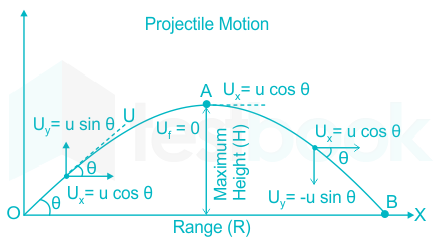Range of projectile:

• The horizontal range of a projectile is the distance along the horizontal plane it would travel, before reaching the same vertical position as it started from.

Formulae in projectile motion:

$$Range\;of\;projectile = \frac{{{u^2}\sin 2θ }}{g}$$

$$Total\;time\;of\;flight = \frac{{2u\;sinθ }}{g}$$

$$Maximum\;Height = \frac{{{u^2}{{\sin }^2}θ }}{{2g}}$$

where u = projected speed, θ = angle at which an object is thrown from the ground and g = acceleration due to gravity = 9.8 m/s2.

Calculation:

Given:

Range of a Projectile motion is given by (R):

$$R= \frac{{{u^2}\sin 2θ }}{g}$$

For horizontal distance to be maximum:

sin 2θ = 1

∴ sin 2θ = sin 90°

∴ θ = 45°.

∴ to take the longest possible jump, an athlete should make an angle of 45° with the ground.

#### Engineering Mechanics MCQ Question 22

A weight of 500 N is supported by two metallic ropes as shown in the figure. The values of tensions T1 and T2 are respectively: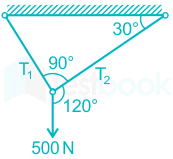1. 433 N and 250 N
2. 250 N and 433 N
3. 353.5 N and 250 N
4. 250 N and 353.5 N

Option 1 : 433 N and 250 N

#### Engineering Mechanics MCQ Question 22 Detailed Solution

Concept:

Lami's Theorem: It is an equation that relates the magnitude of the three co-planner, concurrent and non-collinear forces that keeps a body in equilibrium. It states that each force is proportional to the sine of the angle between the other two forces.Calculation: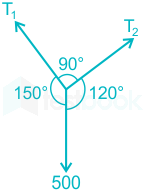$$\frac{{{{\rm{T}}_1}}}{{\sin 120^\circ }} = \frac{{{{\rm{T}}_2}}}{{\sin 150^\circ }} = \frac{{500}}{{\sin 90^\circ }}$$

T1 = 500 × sin 120° and  T2 = 500 sin 150°

T1 = 433 N and T2 = 250 N

#### Engineering Mechanics MCQ Question 23

Which among the following is Not an application of Newton’s third Law of Motion?

1. A man walking on the ground
2. Rowing a boat
3. A fielder pulling his hand backward while catching a ball
4. Bouncing of Ball

Option 3 : A fielder pulling his hand backward while catching a ball

#### Engineering Mechanics MCQ Question 23 Detailed Solution

A fielder pulling his hand backward while catching a ball is an application of newton’s second law of motion.

Newton's Second Law of motion states that the rate of change of momentum of an object is proportional to the applied force in the direction of the force. ie., F=ma. Where F is the force applied, m is the mass of the body, and a is the acceleration produced.

Newton's Third Law of Motion states that 'To every action there is an equal and opposite reaction'.

A fielder pulls his hand backward; while catching a cricket ball coming with a great speed, to reduce the momentum of the ball with a little delay. According to Newton's Second Law of Motion; rate of change of momentum is directly proportional to the force applied in the direction.

#### Engineering Mechanics MCQ Question 24

When a weight of 500 N falls on a spring of stiffness 0.5 kN/m from a height of 2 m. What is the maximum deflection caused in the first fall?

1. 2 m
2. 4 m
3. 1 m
4. 0.63 m

Option 1 : 2 m

#### Engineering Mechanics MCQ Question 24 Detailed Solution

Concept:

If we neglect the air resistance then,

By using energy conservation,

(K + P)I, system = ( K + P)f , system       …(1)

Where, K, P are representing kinetic energy and potential energy. The system includes spring and mass

Taking the reference line as shown in the figure,

$$⇒ {\bf{mgh}} + 0 = \frac{1}{2}{\bf{k}}{{\bf{x}}^2} + {\bf{mg}}\left( { - {\bf{x}}} \right)~$$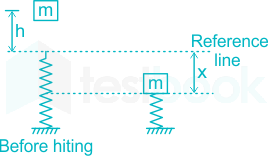∵ mass after hitting comes below the reference line by maximum compression (x) so potential energy for the mass will be taken as with (-) sign. Also, at extreme compression of the spring, the Kinetic energy of the mass will be zero.

$$⇒ {\rm{mg}}\left( {{\rm{h}} + {\rm{x}}} \right) = \frac{1}{2}{\rm{k}}{{\rm{x}}^2}~$$

where m = mass, h = height, k = spring constant, x = maximum deflection in the spring

Calculation:

Given:

Weight (W) = 500 N; h = 2 m; k = 0.5 kN/m = 500 N/m

Weight (W) = mg = 500 N

$$⇒ 500 \times \left( {2\ +\ x} \right) = \frac{1}{2} \times 500 \times {x^2}$$

⇒ x = 3.24 m

Hence the deflection caused in the first fall will be 3.24 m.Mistake PointsThe exact answer to this question is 3.24m, but 3.24 m is not available in the options, this is the official of ISRO LPSC Technical Assistant and they have given the correct answer 2 m.

So, we have to neglect the change in potential energy due to the deflection of the spring.

Neglecting the potential energy change due to the deflection of the spring, we will get the expression:

$$⇒ mgh= \frac{1}{2}{\rm{k}}{{\rm{x}}^2}~$$

$$⇒ 500 \times \left( {2} \right) = \frac{1}{2} \times 500 \times {x^2}$$

⇒ x = 2 m

Hence the approximated deflection caused in the first fall will be 2 m.

#### Engineering Mechanics MCQ Question 25

The kinetic energy of a body is stated to increase by 300 percent. The corresponding increase in the momentum of the body will be ____%

1. 50
2. 100
3. 200
4. 300

Option 2 : 100

#### Engineering Mechanics MCQ Question 25 Detailed Solution

CONCEPT:

• Kinetic energy (K.E): The energy possessed by a body by the virtue of its motion is called kinetic energy.

The expression for kinetic energy is given by:

$$KE = \frac{1}{2}m{v^2}$$

Where m = mass of the body and v = velocity of the body

• Momentum (p): The product of mass and velocity is called momentum.

Momentum (p) = mass (m) × velocity (v)

The relationship between the kinetic energy and Linear momentum is given by:

As we know,

$$KE = \frac{1}{2}m{v^2}$$

Divide numerator and denominator by m, we get

$$KE = \frac{1}{2}\frac{{{m^2}{v^2}}}{m} = \frac{1}{2}\frac{{\;{{\left( {mv} \right)}^2}}}{m} = \frac{1}{2}\frac{{{p^2}}}{m}\;$$ [p = mv]

$$\therefore KE = \frac{1}{2}\frac{{{p^2}}}{m}\;$$

$$p = \sqrt {2m(KE)}$$

CALCULATION:

Let initial Kinetic energy = KE1 = E

Given that:

Final kinetic energy (K.E2) = K.E1 + 300 % of KE1 = E + 3E = 4E

The relation between the momentum and the kinetic energy is given by:

$$P = \sqrt {2m\;(KE)}$$

Final momentum (P') will be:

$$P' = \sqrt {2m\;(KE)_2} = \sqrt {2m\; × 4E} = 2 \sqrt {2m\;E} = 2 P$$

Increase in momentum (ΔP) = P' - P = 2P - P = P

% Increase = $$\frac{\Delta P}{P} \times 100=\frac{2P-P}{P} \times 100=100$$